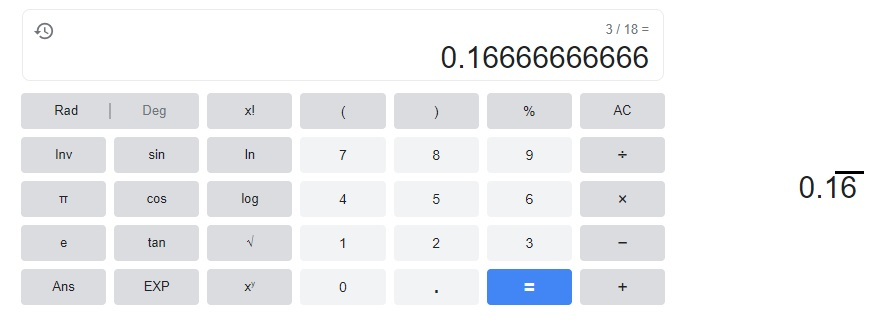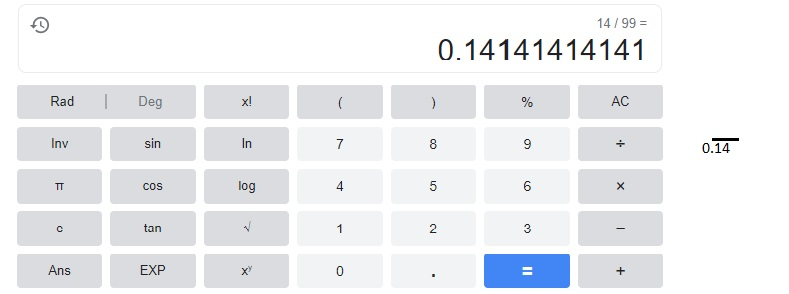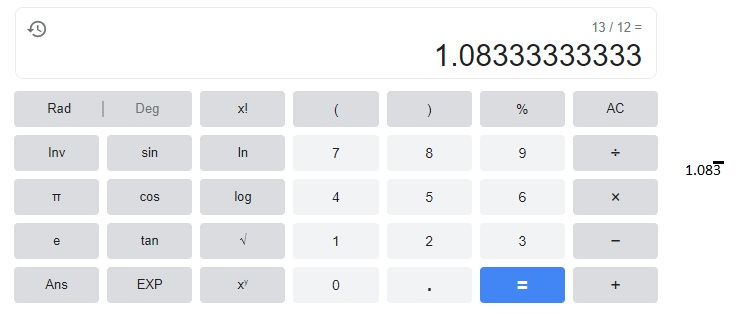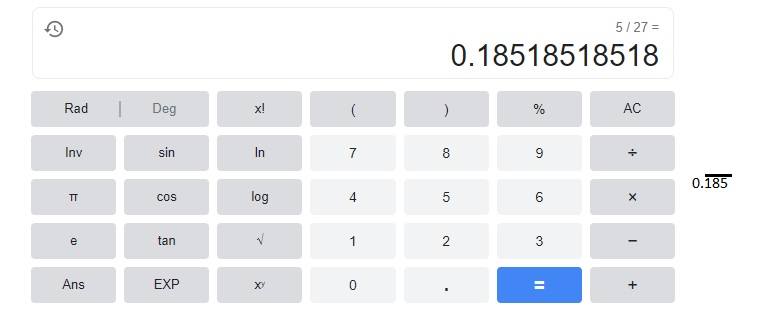# Math in Focus Grade 8 Chapter 3 Answer Key Algebraic Linear Equations

Go through the Math in Focus Grade 8 Workbook Answer Key Chapter 3 Algebraic Linear Equations to finish your assignments.

## Math in Focus Grade 8 Course 3 A Chapter 3 Answer Key Algebraic Linear Equations

### Math in Focus Grade 8 Chapter 3 Quick Check Answer Key

Explain why each pair of equations is equivalent or not equivalent.

Question 1.
x + 4 = 10 and x – 1 = 3
Not equivalent,

Explanation:
Given x + 4 = 10 and x-1 =3 means x = 3+1 =4,
if we substitute x = 4 in x + 4 = 4 + 4 = 8 ≠ 10,
so equations are not equivalent.

Question 2.
$$\frac{1}{5}$$x = 4 and x = 20
Equivalent,

Explanation:
Given $$\frac{1}{5}$$x = 4 and x = 20 upon solving
$$\frac{1}{5}$$x = 4 we get x = 4 X 5 = 20 therefore
pair is equivalent as x = 20.

Question 3.
0.5x + 1 = 1.5x and 2x = 2
Equivalent,

Explanation:
Given 0.5x +1 = 1.5x and 2x =2 means x= 2/2 equal to 1,
so 0.5 (1) + 1 = 1.5 X 1 is 0.5 +1 = 1.5 , So 1.5 = 1.5,therefore
both equations are equivalent.

Question 4.
2(x + 9) = 14 and 2(x – 7) = -18
Equivalent,

Explanation:
Given 2(x -7) = -18 upon solving
2x – 14 = -18,
2x = -18+14,
2x = -4 therfore x = -4/2 = -2 now substituting
2(-2 -7) = – 4 -14 = -18 which is equal to – 18
So both equations are equivalent.

Write a linear equation for each situation. State the independent and dependent variables for each equation.

Question 5.
A manufacturer produces beverages in small and large bottles.
Each small bottle contains s liters of beverages.
Each large bottle contains t liters, which is 1 more liter than the
quantity in the small bottle. Express t in terms of s.
t = (s + (sX1)) liters,

Explanation:
Given A manufacturer produces beverages in small and large bottles.
Each small bottle contains s liters of beverages.
Each large bottle contains t liters, which is 1 more liter than the
quantity in the small bottle. Expressing t in terms of s as t = (s + (sX1))  liters.

Question 6.
Hazel is 4 years younger than Alphonso. Express Alphonso’s age, a, in terms of Hazel’s age, h.
h=(a-4),

Explanation:
Given Hazel is 4 years younger than Alphonso.
Expressing Alphonso’s age, a, in terms of Hazel’s age, h.
h=(a-4).

Question 7.
A bouquet of lavender costs $12. Find the cost, C, of n bouquets of lavender. Answer: C =$12n

Explanation:
Given a bouquet of lavender costs $12, the cost, C, of n bouquets of lavender is c =$12 X n = \$12n.

Question 8.
The distance, d miles, traveled by a bus is 40 times the time,t hours,
used for the journey. Find d in terms of t.
d = 40t,

Explanation:
Given the distance, d miles, traveled by a bus is 40 times the time,t hours,
used for the journey is d = 40t.

Solve each equation.

Question 9.
4x = 14 + 2x
x =7,

Explanation:
Given 4x = 14 + 2x, upon solving
4x – 2x = 14,
2x = 14,
x = 14/2 = 7.

Question 10.
$$\frac{1}{3}$$v = 2 – $$\frac{2}{9}$$v
v = $$\frac{18}{5}$$,

Explanation:
Given $$\frac{1}{3}$$v = 2 – $$\frac{2}{9}$$v,
$$\frac{1}{3}$$v = $$\frac{18 -2v}{9}$$,
$$\frac{9}{3}$$v = 18 -2v,
3 v = 18 -2v,
5v = 18, therefore v = $$\frac{18}{5}$$.

Question 11.
c + 2(1 – c) = 10 – 3c
c = 4,

Explanation:
Given c + 2(1 – c) = 10 – 3c,
c + 2 – 2c = 10 -3c,
2 – c = 10 – 3c,
3c-c = 10-2,
2c = 8,
c = 4.

Question 12.
3(2 + 3x) = 13(x + 2)
x = -5,

Explanation:
Given 3(2+3x) = 13(x +2) upon solving
6 + 9x = 13x + 26,
6-26 = 13x – 9x,
-20 = 4 x,
x = -5.

Write the decimal for each fraction. Use bar notation.

Question 13.
$$\frac{3}{18}$$Explanation:
A bar notation, which is a way to show repeating numbers after a decimal point,
here when 3 divides by 18 we get 0.166666 where 6 is repeating so we keep a bar on 6
as shown above.

Question 14.
$$\frac{14}{99}$$Explanation:
When 14 divides by 99 we get 0.14141414 where 14 is repeating so we keep a bar on 14
as shown above.

Question 15.
$$\frac{13}{12}$$$$\frac{5}{27}$$